SPSS TUTORIALS BASICS DATA ANALYSIS CHI-SQUARE TESTS ANOVA T-TESTS

# Linear Regression in SPSS – A Simple Example

A company wants to know how job performance relates to IQ, motivation and social support. They collect data on 60 employees, resulting in job_performance.sav. Part of these data are shown below.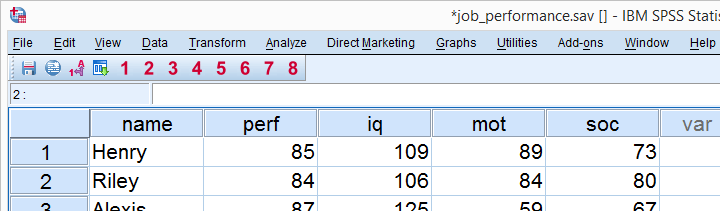## Quick Data Check

We usually start our analysis with a solid data inspection. Since that's already been done for the data at hand, we'll limit it to a quick check of relevant histograms and correlations. The syntax below shows the fastest way to generate histograms.

## Syntax for Running Histograms

*Inspect histograms for all regression variables.

frequencies perf to soc
/format notable
/histogram.

## Histograms Output

We'll show the first histogram below. Note that each histogram is based on 60 observations, which corresponds to the number of cases in our data. This means that we don't have any system missing values.
Second, note that all histograms look plausible; none of them have weird shapes or extremely high or low values. As we see, histograms provide a very nice and quick data check.## Running the Correlation Matrix

Next, we'll check whether the correlations among our regression variables make any sense. We'll create the correlation matrix by running correlations perf to soc.

## Inspecting the Correlation Matrix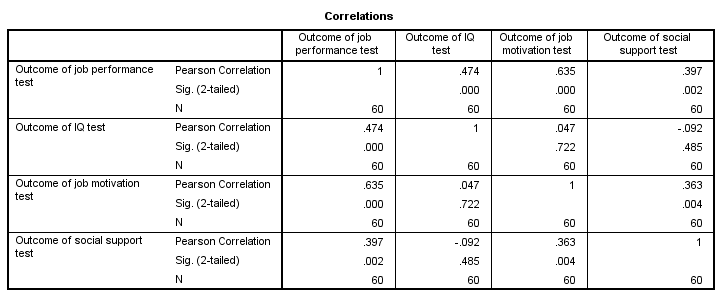Most importantly, the correlations are plausible; job performance correlates positively and substantively with all other variables. This makes sense because each variable reflects as positive quality that's likely to contribute to better job performance.
Note that IQ doesn't really correlate with anything but job performance. Perhaps we'd expect somewhat higher correlations here but we don't find this result very unusual. Finally, note that the correlation matrix confirms that there's no missing values in our data.

## Linear Regression in SPSS - Model

We'll try to predict job performance from all other variables by means of a multiple regression analysis. Therefore, job performance is our criterion (or dependent variable). IQ, motivation and social support are our predictors (or independent variables). The model is illustrated below.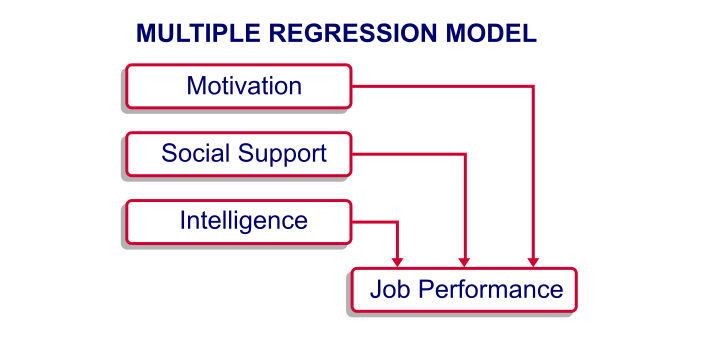A basic rule of thumb is that we need at least 15 independent observations for each predictor in our model. With three predictors, we need at least (3 x 15 =) 45 respondents. The 60 respondents we actually have in our data are sufficient for our model.

## Linear Regression in SPSS - Purpose

Keep in mind that regression does not prove any causal relations from our predictors on job performance. However, we do find such causal relations intuitively likely. If they do exist, then we can perhaps improve job performance by enhancing the motivation, social support and IQ of our employees.
If there aren't any causal relations among our variables, then being able to predict job performance may still be useful for assessing job applicants; we can measure their IQ, motivation and social support but we can't measure their job performance before we actually hire them.

## Running our Linear Regression in SPSS

The screenshots below illustrate how to run a basic regression analysis in SPSS.In the linear regression dialog below, we move perf into the Dependent box. Next, we move IQ, mot and soc into the Independent(s) box. Clicking results in the next syntax example.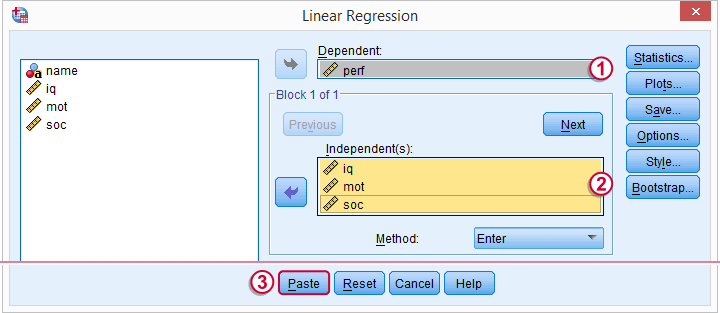## Linear Regression in SPSS - Syntax

*SPSS regression with default settings.

REGRESSION
/MISSING LISTWISE
/STATISTICS COEFF OUTS R ANOVA
/CRITERIA=PIN(.05) POUT(.10)
/NOORIGIN
/DEPENDENT perf
/METHOD=ENTER iq mot soc.

## Linear Regression in SPSS - Short Syntax

We can now run the syntax as generated from the menu. However, we do want to point out that much of this syntax does absolutely nothing in this example. Running regression/dependent perf/enter iq mot soc. does the exact same things as the longer regression syntax.

## SPSS Regression Output - Coefficients Table

SPSS regression with default settings results in four tables. The most important table is the last table, “Coefficients”.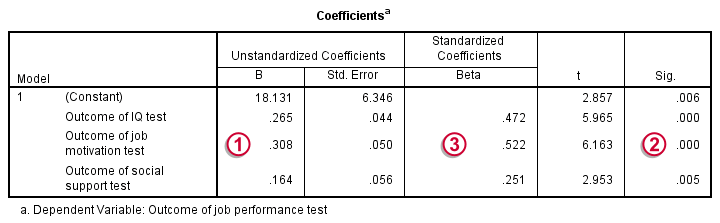The b coefficients tell us how many units job performance increases for a single unit increase in each predictor. Like so, 1 point increase on the IQ tests corresponds to 0.27 points increase on the job performance test. Given only the scores on our predictors, we can predict job performance by computing Job performance = 18.1 + (0.27 x intelligence) + (0.31 x motivation) +
(0.16 x social support)
Importantly, note that all b coefficients are positive numbers; higher IQ is associated with higher job performance and so on. B coefficients having the “wrong direction” often indicate a problem with the analysis known as multicollinearity.The column “Sig.” holds the p-values for our predictors. As a rule of thumb, we say that a b coefficient is statistically significant if its p-value is smaller than 0.05. All of our b coefficients are statistically significant.The beta coefficients allow us to compare the relative strengths of our predictors. These are roughly 2 to 2 to 1 for IQ, motivation and social support.

## SPSS Regression Output - Model Summary Table

The second most important table in our output is the Model Summary as shown below.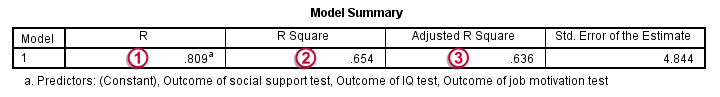As we previously mentioned, our model predicts job performance. R denotes the correlation between predicted and observed job performance. In our case, R = 0.81. Since this is a very high correlation, our model predicts job performance rather precisely.r square is simply the square of R. It indicates the proportion of variance in job performance that can be “explained” by our three predictors.Because regression maximizes R square for our sample, it will be somewhat lower for the entire population, a phenomenon known as shrinkage. The adjusted r-square estimates the population R square for our model and thus gives a more realistic indication of its predictive power.

## SPSS Linear Regression - Conclusion

The high adjusted R squared tells us that our model does a great job in predicting job performance. On top of that, our b coeffients are all statistically significant and make perfect intuitive sense. Mission accomplished.
We should add, however, that this tutorial illustrates a problem free analysis on problem free data. When applying regression analysis to more difficult data, you may encounter complications such as multicollinearity and heteroscedasticity. These are beyond the scope of this basic regression example. However, we'll cover such specialist topics in our future tutorials.

# Tell us what you think!

*Required field. Your comment will show up after approval from a moderator.

# THIS TUTORIAL HAS 48 COMMENTS:

•### By edward m james on August 12th, 2020

I have a problem with 10000 lines of data,10 variables and 5 algorithm solutions expected. 4 variables are non continuous. How many runs needed to find solutions. What is total run time?

•### By ANUNAOBI JAMES on August 19th, 2020

I need a practical application of regression analyses in project work. Chapter four presentation precisely

•### By Rosemary Guvhu on December 6th, 2020

Well explained Very useful source